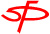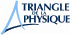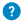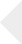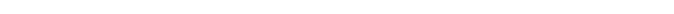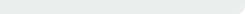Characteristic lengths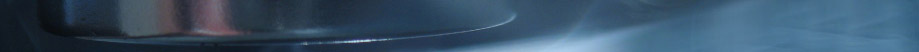# Characteristic

## Lengths

A superconductor is defined by many physical quantities, the same way a human being is defined by weight, size and hair colour. These are the most important ones:

### The critical temperature Tc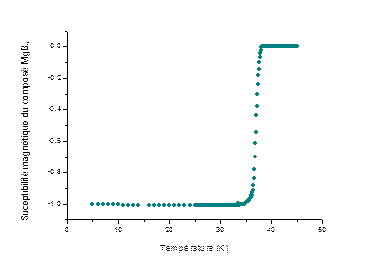It corresponds to the temperature below which the material becomes superconducting. We can use degrees Celsius but physicists prefer degrees Kelvin (just add 273 to the temperature in degrees Celsius).

### The critical current Ic

It corresponds to the maximum current that can go through a superconductor. If the current exceeds this value, the material becomes a normal metal again and starts resisting and heating like any other metal. More often, physicists use the term “current density” (jc), that is the current divided by the cross section of the electric wire.  For instance, in a cuprate, the current density is more than 10 000 amperes per cm2.

### The critical field Bc

It corresponds to the maximum magnetic field that can be applied to a superconductor. Above the critical field Bc, the material becomes a normal metal again. For instance, in mercury, this field is 0.04 Tesla (about 1000 times the earth magnetic field. For some superconductors, we have to define two critical fields, Bc1 and Bc2, because of the presence of vortices. For example, in a cuprate, Bc1 is about 0.01 Tesla, but Bc2 can be as high as 60 teslas, which is more than a million times higher than the Earth’s magnetic field

### The penetration length λ ("lambda")

When a magnetic field is applied to a superconductor, it is expelled and can only penetrate a short depth of the surface of the sample, measured as the penetration length. It is usually very small, from 10 to 100 nanometres (1 nanometre = 1 billionth of a metre).

### The coherence length ξ ("xi")

It corresponds to the distance to which a Cooper pair spreads, or to the necessary distance for a superconducting wave to develop. It also corresponds to the size of the heart of the vortex. It can vary highly depending on the superconducting material, going from a micrometre in superconductors such as aluminium to only a few nanometres in cuprates.

### The gap

The superconductor is characterized by its wavefunction formed from the Cooper pairs. This wavefunction has an energy called “gap”: it corresponds to the minimum energy needed to break one of the Cooper pairs. In conventional superconductors, the higher the Tc, the  greater the gap, and the latter is the same in all directions (isotropic). In unconventional superconductors such as cuprates or heavy fermions, the gap can be “anisotropic” and can present symmetries with very different shapes.